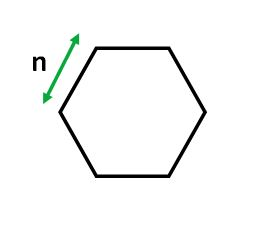Open in App
Not now

# Area of a Hexagon

• Difficulty Level : Basic
• Last Updated : 26 Dec, 2022

A hexagon is a 6-sided, 2-dimensional geometric figure. The total of the internal angles of any hexagon is 720°. A regular hexagon has 6 rotational symmetries and 6 reflection symmetries. All internal angles are 120 degrees.Examples :

```Input: 4
Output: 41.5692

Input: 6
Output: 93.5307```

Number of vertices: 6
Number of edges: 6
Internal angle: 120°
Area = (3 √3(n)2 ) / 2

How does the formula work? There are mainly 6 equilateral triangles of side n and area of an equilateral triangle is (sqrt(3)/4) * n * n. Since in hexagon, there are total 6 equilateral triangles with side n, area of the hexagon becomes (3*sqrt(3)/2) * n * n

## C++

 `// CPP program to find ` `// area of a Hexagon` `#include ` `#include ` `using` `namespace` `std;`   `// function for calculating` `// area of the hexagon.` `double` `hexagonArea(``double` `s)` `{` `    ``return` `((3 * ``sqrt``(3) * ` `            ``(s * s)) / 2);     ` `}`   `// Driver Code` `int` `main()` `{` `    ``// Length of a side ` `    ``double` `s = 4; ` `    ``cout << ``"Area : "` `         ``<< hexagonArea(s);` `    ``return` `0;` `}`

## Java

 `import` `java.io.*;` `public` `class` `GFG ` `{ ` `    ``// Create a function for calculating` `    ``// the area of the hexagon.` `    ``public` `static` `double` `hexagonArea(``double` `s) ` `    ``{` `        ``return` `((``3` `* Math.sqrt(``3``) * ` `                ``(s * s)) / ``2``);` `    ``} ` `        `  `    ``// Driver Code` `    ``public` `static` `void` `main(String[] args) ` `    ``{     ` `        ``// Length of a side` `        ``double` `s = ``4``;      ` `        ``System.out.print(``"Area: "` `+ ` `                          ``hexagonArea(s) );` `    ``}` `}`

## Python3

 `# Python3 program to find` `# area of a Hexagon` `import` `math`   `# Function for calculating ` `# area of the hexagon.` `def` `hexagonArea(s):` `    `  `    ``return` `((``3` `*` `math.sqrt(``3``) ``*` `            ``(s ``*` `s)) ``/` `2``); ` `    `  `# Driver code     ` `if` `__name__ ``=``=` `"__main__"` `: `   `    ``# length of a side. ` `    ``s ``=` `4`   `    ``print``(``"Area:"``,``"{0:.4f}"` `. ` `           ``format``(hexagonArea(s)))`   `# This code is contributed by Naman_Garg`

## C#

 `// C# program to find` `// area of a Hexagon` `using` `System;`   `class` `GFG ` `{` `    `  `    ``// Create a function for calculating` `    ``// the area of the hexagon.` `    ``public` `static` `double` `hexagonArea(``double` `s) ` `    ``{` `        ``return` `((3 * Math.Sqrt(3) * ` `                ``(s * s)) / 2);` `    ``} ` `        `  `    ``// Driver Code` `    ``public` `static` `void` `Main() ` `    ``{` `        ``// Length of a side ` `        ``double` `s = 4; ` `        `  `        ``Console.WriteLine(``"Area: "` `+ ` `                           ``hexagonArea(s) );` `    ``}` `}`   `// This code is contributed by vt_m.`

## PHP

 ``

## Javascript

 ``

Output :

`Area: 41.5692`

Time Complexity: O(1)
Auxiliary Space: O(1), since no extra space has been taken.

My Personal Notes arrow_drop_up
Related Articles